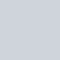# 獲取當前時間

``````import time
now = time.localtime()
``````

# 格式化時間

``````time_str = time.strftime("%Y-%m-%d %H:%M:%S", now)
``````

# 範例程式碼

``````import time

now = time.localtime()
time_str = time.strftime("%Y-%m-%d %H:%M:%S", now)

print(time_str)
``````